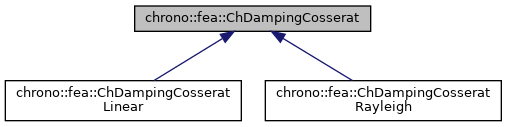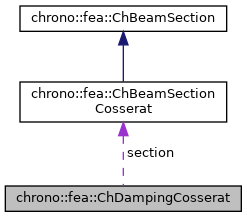chrono::fea::ChDampingCosserat Class Referenceabstract

## Description

Base interface for structural damping of beam sections of Cosserat type, where xyz force "n" and xyz torque "m" are a 6-dimensional function of generalized strain speeds, "e'" traction/shear speed and "k'" curvature speed, as: {n,m}=f({e',k'}) Children classes implement this function in different ways.

#include <ChBeamSectionCosserat.h>

Inheritance diagram for chrono::fea::ChDampingCosserat:[legend]
Collaboration diagram for chrono::fea::ChDampingCosserat:[legend]

## Public Member Functions

virtual void ComputeStress (ChVector<> &stress_n, ChVector<> &stress_m, const ChVector<> &dstrain_e, const ChVector<> &dstrain_k)=0
Compute the generalized cut force and cut torque, caused by structural damping, given actual deformation speed and curvature speed. More...

virtual void ComputeDampingMatrix (ChMatrixDynamic<> &R, const ChVector<> &dstrain_e, const ChVector<> &dstrain_k)
Compute the 6x6 tangent material damping matrix, ie the jacobian [Rm]=dstress/dstrainspeed. More...

virtual void SetAsRectangularSection (double width_y, double width_z)=0
Shortcut: set parameters at once, given the y and z widths of the beam assumed with rectangular shape.

virtual void SetAsCircularSection (double diameter)=0
Shortcut: set parameters at once, given the diameter of the beam assumed with circular shape.

## Public Attributes

ChBeamSectionCosseratsection

## ◆ ComputeDampingMatrix()

 void chrono::fea::ChDampingCosserat::ComputeDampingMatrix ( ChMatrixDynamic<> & R, const ChVector<> & dstrain_e, const ChVector<> & dstrain_k )
virtual

Compute the 6x6 tangent material damping matrix, ie the jacobian [Rm]=dstress/dstrainspeed.

This must be overridden by subclasses if an analytical solution is known (preferred for high performance), otherwise the base behaviour here is to compute [Rm] by numerical differentiation calling ComputeStress() multiple times.

Parameters
 R 6x6 material stiffness matrix values here dstrain_e current strain speed (deformation part) dstrain_k current strain speed (curvature part)

Reimplemented in chrono::fea::ChDampingCosseratLinear.

## ◆ ComputeStress()

 virtual void chrono::fea::ChDampingCosserat::ComputeStress ( ChVector<> & stress_n, ChVector<> & stress_m, const ChVector<> & dstrain_e, const ChVector<> & dstrain_k )
pure virtual

Compute the generalized cut force and cut torque, caused by structural damping, given actual deformation speed and curvature speed.

This MUST be implemented by subclasses.

Parameters
 stress_n local stress (generalized force), x component = traction along beam stress_m local stress (generalized torque), x component = torsion torque along beam dstrain_e local strain speed (deformation); x elongation speed; y,z shear speeds dstrain_k local strain speed (curvature); x torsion speed; y, z line curvature speeds

Implemented in chrono::fea::ChDampingCosseratLinear.

The documentation for this class was generated from the following files:
• /builds/uwsbel/chrono/src/chrono/fea/ChBeamSectionCosserat.h
• /builds/uwsbel/chrono/src/chrono/fea/ChBeamSectionCosserat.cpp